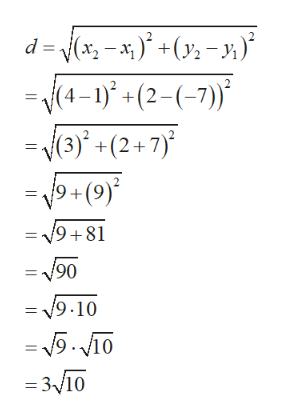Question
6 views

Find the distance between the points (1, −7) and (4, 2).

check_circle

Step 1

To find the distance between the given points

Step 2

To find the distance between the point, we use the distance formula connecting the endpoints (x1, y1) and (x2,y2) of line shown below:

Step 3

Now in order to find the distance between the given points, we will plug (x1, y1)=(1, -7) and (x2,y2)...help_outlineImage Transcriptionclosed = j(x, - x; )* +(y2 - »)* -(1-1)" + (2-(-7)* = V(3)*. +(2+7)° = /9+(9)' = 19+81 V90 = 19.10 = 5. V10 = 3V10 fullscreen

### Want to see the full answer?

See Solution

#### Want to see this answer and more?

Solutions are written by subject experts who are available 24/7. Questions are typically answered within 1 hour.*

See Solution
*Response times may vary by subject and question.
Tagged in

### Algebra